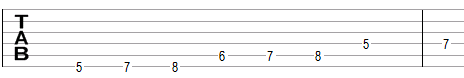# Minor gypsy scale

The minor gypsy scale is a heptatonic scale that can be built using the following intervals between notes: 1, ½, 1½, ½, ½, 1, 1.

One example of the minor gypsy scale is A, B, C, D#, E, F, G. This example is shown below in traditional notation and in guitar tablature notation.One way to think about this scale is to take the natural minor scale and raise the fourth degree of the scale by one semitone. The example above is the natural minor scale in A (A, B, C, D, E, F, G), but with the raised fourth D#.

Another way to think about this scale is to take the Phrygian scale and to raise the seventh degree of the scale. One example of the Phrygian is E, F, G, A, B, C, D and raising the seventh produces E, F, G, A, B, C, D#. The Phrygian is common in flamenco, where alternating between a normal and a raised third (G and G# in this example) and a normal and a raised seventh (D and D# in this example) is common. Raising the third in this example produces E, F, G#, A, B, C, D, which is an example of the Spanish gypsy scale, which is also the fifth mode of the Harmonic minor scale. Raising the seventh produces the minor gypsy scale described here. Raising both the third and the seventh produces the Hungarian scale, also known as Egyptian scale, Arabic scale, double harmonic major scale, or simply gypsy scale.

## Modes of the minor gypsy scale

The minor gypsy scale has no commonly used modes, except the scale itself.

## Three-note chords on the minor gypsy scale

The following are common triads built on the notes of the minor gypsy scale.

• On the root of the scale (on the tonic): minor chord (e.g., Am composed of A, C, E), diminished chord (Adim = A, C, D#) or suspended chord (Asus2 = A, B, E).
• On the second note (on the supertonic): augmented chord (Baug = B, D#, G).
• On the third note (on the mediant): major chord (C = C, E, G), minor chord (C = C, D#, G), or suspended chord (Csus4 = C, F, G).
• On the fourth note (on the subdominant): augmented chord (D#aug = D#, G, B).
• On the fifth note (on the dominant): minor chord (Em = E, G, B) or suspended chord (Esus4 = E, A, B).
• On the sixth note (on the submediant): major chord (F = F, A, C) or suspended chord (Fsus2 = F, G, C).
• On the seventh note (on the leading tone: major chord (Gaug = G, B, D#).

## Four-note chords on the minor gypsy scale

The following are seventh chords built on the notes of the gypsy scale.

• On the first note: minor seventh chord (e.g. Amin7 composed of A, C, E, G) or half-diminished seventh chord (minor seventh chord with a flat fifth, Am7b5 = A, C, D#, G).
• On the second note: augmented seventh chord (B7#5 = B, D#, G, A).
• On the third note: major seventh chord (Cmaj7 = C, E, G, B) or minor-major seventh chord (Cmmaj7 = C, D#, G, B).
• On the fourth note: there are no common seventh chords.
• On the fifth note: minor-major seventh chord (Emmaj7 = E, G, B, D#).
• On the sixth note: major seventh chord (Fmaj7 = F, A, C, E) or dominant seventh chord (F7 = F, A, C, D#).
• On the seventh note: augmented seventh chord (G7#5 = G, B, D#, F).

## Intervals on the minor gypsy scale

The minor gypsy scale is composed of the following intervals.

• A major second, e.g., the interval between A and B is equal to two semitones.
• A minor third, e.g., the interval between A and C is equal to three semitones.
• An augmented fourth, e.g., the interval between A and D# is equal to six semitones.
• A perfect fifth, e.g., the interval between A and E is equal to seven semitones.
• A minor sixth, e.g., the interval between A and F is equal to eight semitones.
• A minor seventh, e.g., the interval between A and G is equal to ten semitones.

Scale, Scale (index)

Super

### Filtered HTML

• Freelinking helps you easily create HTML links. Links take the form of [[indicator:target|Title]]. By default (no indicator): Click to view a local node.